Home PDVL SHP (Arduissimo) (Wave-pipelining) Dynamic DFT failures about

C-Slow Retiming

It must be stated, that C-Slow Retiming (CSR) is already very well known. Nevertheless, I do think, that this technique has much more potential than it is currently used. This CloudX initiative is set up to change this and to place real-world examples on the table of anybody who asks for it.

Theory of CSR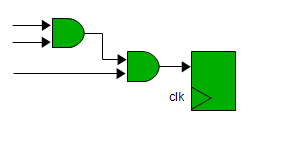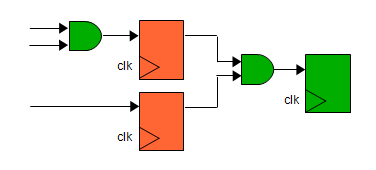Figure 1: Solving an equation in one cycle. Figure 2: Solving the same equation in 2 cycles.

Figure 1 shows a simplified example of a digital logic. The combinatorial logic is solved within one cycle. In Figure 2 registers are inserted in the digital logic. The logic result is the same, but it takes now 2 cycles so solve the same combinatorial logic. The point is, that in the second cycle, you can already start a completely independent calculation of a new result. The simplified example also shows, that in theory you can run the clock at twice the speed, so that the overall time to solve one single equation does not change. In other words, by adding registers, you have the chance to solve the same equation twice as often.

Now let's use this technique on a complete design.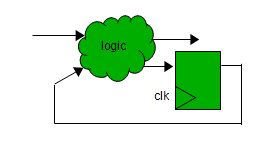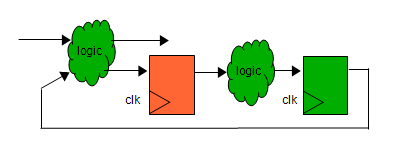Figure 3: Simplification of single clock design. Figure 4: Single clock design after register insertion.

Any (single clock) design can be defined as a set of inputs, outputs, a graph of logic elements and registers (Figure 3). CSR now executes this register insertion on a more complex design automatically, as can be seen in Figure 4. Now is takes 2 cycles to achieve the same behavior as the original design, but you have a second, totally independent design which uses the combinatorial logic in a time sliced fashion.

It is totally irrelevant, if the original design is already pipelined (as in a CPU for instance). If you follow the rule to insert the same number of registers in any of the original logic paths, you multiply the functionality of the design/core. If the registers are timing driven placed, the performance of a single core remains almost the same. More register levels can be inserted and the functionality multiplies accordingly. The automatic register insertion on RTL simplifies the SoC-implementation, necessary code optimizations (e.g. memories) by hand and the verification process.

CSR
and area reduction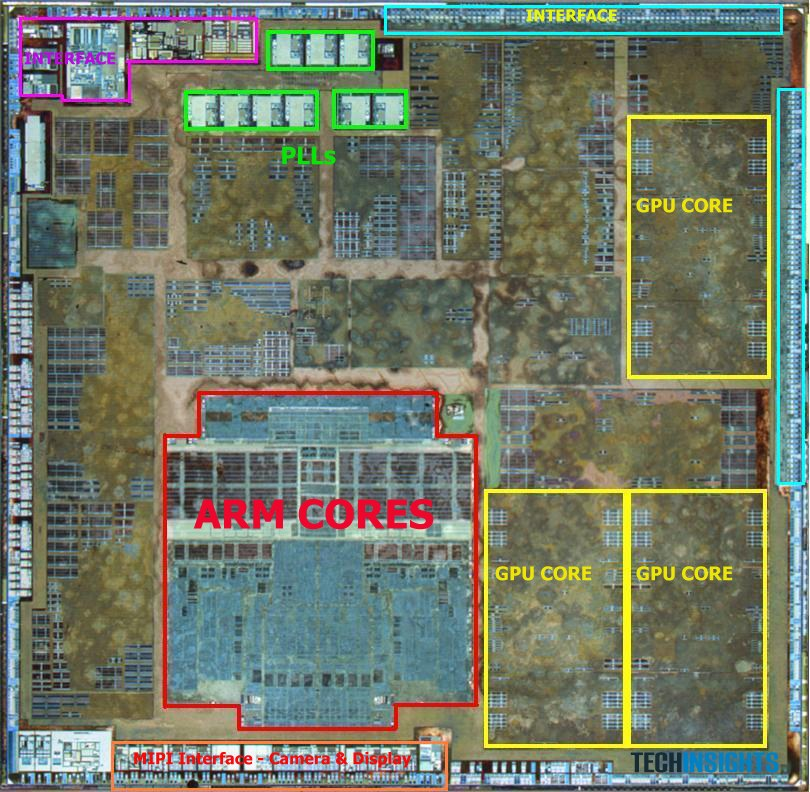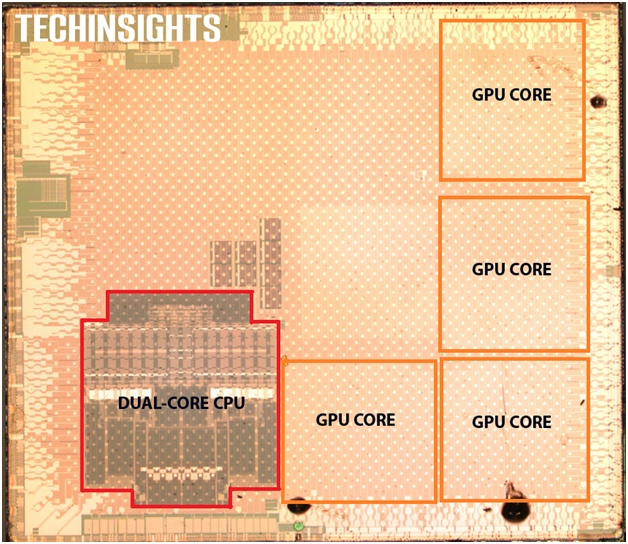Figure 5: A6 processor (source: techinsights.com).          Figure 6: A6X processor (source: techinsights.com).

The most obvious advantage of using CSR is area reduction. CSR can be applied to combinatorial logic of identical core instantiations. As in the case of the A6 processor (Figure 5), CSR could potentially be executed on the two ARM cores (10% area reduction of the red area) as well as on the three GPU cores (32% area reduction of the yellow area). This would already result in a 6.7% area reduction of the complete die.

These numbers might not be overwhelming, but since more and more multicores are implemented - "the processor is the new transistor" - the area reduction might go up to 10%, 20% or even more. In the FPGA world, the area utilization is even greater, since the registers "already exist".

An example for the increasing importance of using CSR is the A6X processor (Figure 6). It is using a larger GPU and now 4 instead of 3. Since CSR is more efficient of larger designs (GPUs) and the number of identical designs increased, the potential area reduction for the A6X is now 14%.

CSR
on RTL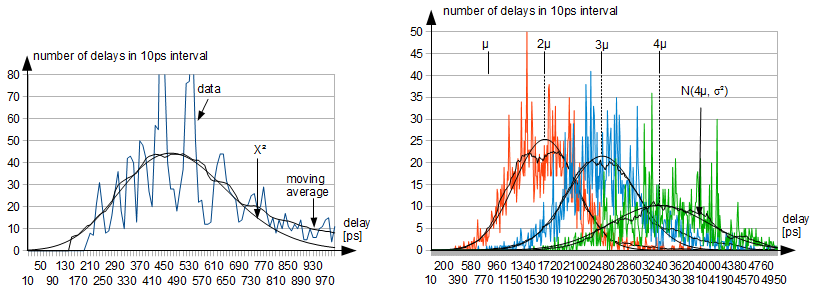Figure 7: X² distribution of single net delay.                    Figure 8: Gaussian for consecutive nets.

CSR on RTL has a lot of advantages, and it is questionable if it is doable on netlist at all. I use simple empirical observations, such as that the net delay of an FPGA net follows a X² distribution (Figure 7), which ultimately leads with k > 70 to a Gaussian for consecutive delays (Figure 8). This observation allows the insertion of registers on RTL quite efficiently .

Examples

Examples (2010) of using CSR can be found here:

Hyper Pipelined Open RISC OR1200 Core, http://opencores.org/project,or1200_hp (Verilog)
Hyper Pipelined AVR Core, http://opencores.org/project,avr_hp (VHDL)

CSR and TMR

CSR can also be used to generate a time multiplexed triple modular redundant system .

References

 T.Strauch, "Timing Driven C-Slow Retiming on RTL for MultiCores on FPGAs", ParaFPGA2013, 10-13 September 2013, Munich, Germany, pages 1-6, also at Cornell University Library, 14th July 2018, https://arxiv.org/abs/1807.05446, or here.

 T.Strauch, "Using C-Slow Retiming in Safety Critical and Low Power Applications", First International Workshop on FPGAs in Aerospace Applications", FASA 2014, 5 September 2014, Munich, Germany, with an almost similar version publicly available here: T.Strauch, "Running Identical Threads in C-Slow Retiming based Designs for Functional Failure Detection", Cornell University Library, 4th February 2015, https://arxiv.org/abs/1502.01237, or here.

Articles

1. T. Strauch, 2010, Hyper pipelining of multicores and SoC interconnects, EETimes, 11/2/2010, link, pdf